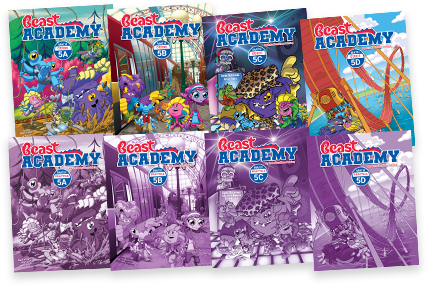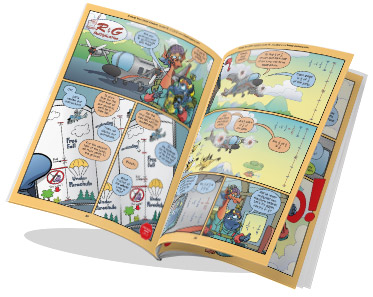By Jason Batterson, Shannon Rogers, Kyle Guillet, and Chris Page
Illustrated by Erich Owen

# Level 5 Math Books

Ages 11‑13By Jason Batterson, Shannon Rogers, Kyle Guillet, and Chris Page
Illustrated by Erich Owen\$118.00

## Level 5 Books Information

Designed in an engaging comic-book style format, our Level 5 curriculum includes the Guide books and Practice books for all four units (5A, 5B, 5C, 5D).

• The Guide book is a full-color paperback textbook, which teaches concepts in a comic-book style.
• The Practice book complements the Guide book and includes 300+ problems ranging from introductory-level exercises to challenging puzzles and word problems.Level 5 has four units: A, B, C and D. Topics build upon each other from unit to unit.

5A
5B
5C
5D
3D Solids
Classifying solids, nets, surface area, and volume.
Statistics
Median, average (mean), weighted averages, range, and mode.
Sequences
Patterns, finding unknown terms, arithmetic sequences, and triangular numbers.
Percents
Converting among fractions, decimals, and percents; estimation; proportions; and percent change.
Integers
Multiplication, long products, division, exponents, and opposites (negation).
Factors & Multiples
Factors, greatest common factor (gcf), multiples, least common multiple (lcm), factorials.
Ratios & Rates
Ratios, proportions, speed, rates, and unit conversions.
Square Roots
Squares, square roots, cube roots, estimation, comparison, and the Pythagorean theorem.
Expressions & Equations
Notation, like terms, simplifying expressions, and solving multi-step equations.
Fractions
Addition and subtraction with like and unlike denominators, multiplication, simplification, division.
Decimals
Powers of 10, multiplication, division, and converting between fractions and decimals.
Exponents
Multiplying and dividing powers, negative exponents, scientific notation, raising a power to a power, and solving equations.

We recommend taking the Level 5A Placement Test to determine if your student is ready to take Beast Academy Level 5.

Solutions to the placement test are found at the end of the test. Your student may also take the Level 5B, 5C, or 5D placement tests to determine readiness per unit.Get the guide books for sure, because they are endearing and interesting and adorable and wonderful and they make your kid fall in love with doing math (even if they already love math).

Kjersti, BA parent